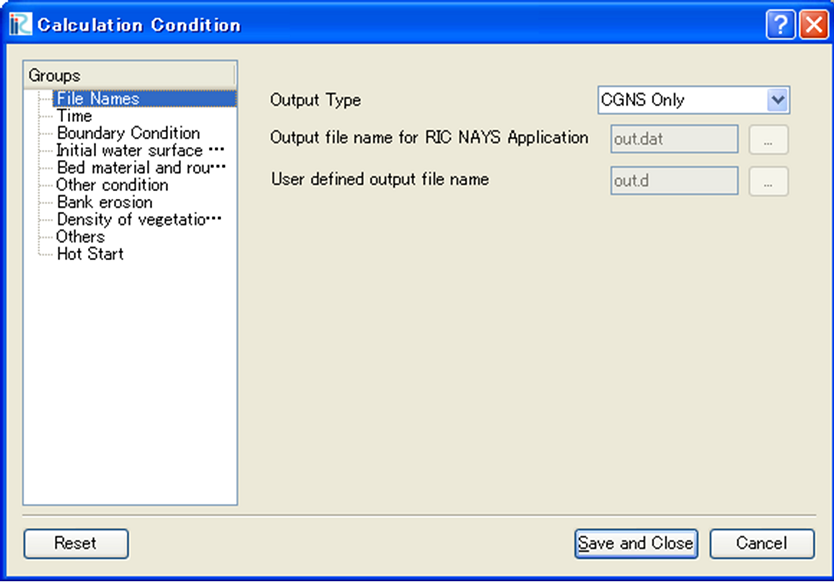# [Calculation Conditions]¶

Below are the functions of items under the [Calculation Conditions] menu.

When the Pre-processor is active, calculation conditions can be set by using the menu in [Calculation Conditions] (C). The function of each menu item is explained here.

## [Setting] (S)¶

Description: Sets the calculation conditions.

When you select this, the [Calculation Conditions] dialog (Figure 374) will open. Set the calculation conditions and click on [Save and Close]. To restore the default calculation conditions, click on [Reset].

The calculation conditions that require setting depends on the solver user uses.Figure 374 The [Calculation Conditions] dialog

## [Import] (I)¶

Description: Imports the calculation conditions.

The function of this item is the same to [Calculation Condition] under [Import] menu under [File] menu. Refer to [Calculation Conditions] (C).

## [Export] (E)¶

Description: Exports the calculation conditions.

The function of this item is the same to [Calculation Condition] under [Export] menu under [File] menu. Refer to [Calculation Conditions] (C).